- Practical Electron Microscopy and Database -
- An Online Book -
Microanalysis | EM Book                                                                                   https://www.globalsino.com/EM/

This book (Practical Electron Microscopy and Database) is a reference for TEM and SEM students, operators, engineers, technicians, managers, and researchers.
=================================================================================

When two oppositely charged ions in solids are bonded together, the bond-length (d) is the sum of the two radii (r+ and r-) of the two different ions as shown in Figure 3138a. Therefore, we can have d = r+ + r-.Figure 3138a. Relations between bond-length and ionic radii.

According to Radius Ratio Rule, we have:
i) As the size (ionic radius) of a cation increases, more anions with a particular size can pack around it.
ii) For a specific structure, the limiting radius ratio can be calculated. The limiting radius ratio is the minimum allowable value for the ratio of ionic radii (ρ=r+/r-) for this structure to be stable. Here, r+ is the radius of the cation and r- is the radius of the surrounding anions. Note that the anions are usually larger than cations.

If all of the atoms in a crystal are the same size, the maximum number of atoms that are coordinated around any individual is 12 (also called 12-fold coordination) in which there are two ways that atoms can be packed. For a single layer of atoms with an equal size, there are two kinds of voids between the atoms as shown in Figure 3138b (a):
i) B voids with triangles pointing up.
ii) C voids with the triangles pointing down.

As shown in Figure 3138b (b), if the second layer of atoms is added and its atoms occupy the space above the B voids and the third layer of atoms is added and its atoms occupy the positions over the C voids in the A layer, this will result in cubic closest packing with a stacking sequence of ABC ABC ABC.... etc. As shown in Figure 3138b (c), if the second layer of atoms is added and its atoms occupy the space above the B voids followed by the third layer above the A atoms, this will result in hexagonal closest packing (HCP) with a stacking sequence of AB AB AB ....etc and with the c-axis perpendicular to the AB AB layers.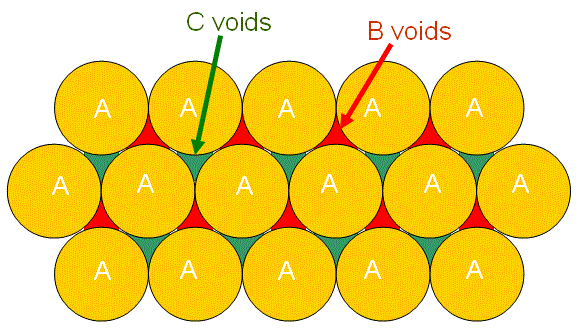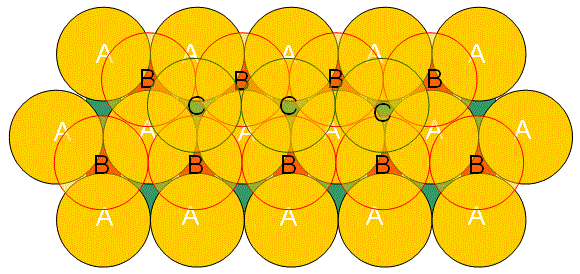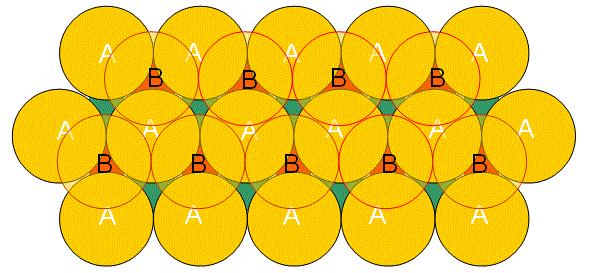(a) (b) (c)

Figure 3138b. (a) Single layer of atoms, (b) Formed cubic closest packing structure, and (c) Formed HCP structure.

In order to allow the surrounding anions to touch each other as well as touch the cation when the size of the cation decreases (r+/r- decreases consequently) the coordination will have 8 anions surrounding the cation. This is called 8-fold coordination or cubic coordination.

If the size of the coordinated cation further becomes smaller, then it will become too small to touch the surrounding anions because there is limiting radius ratio when the r+/r- becomes too small as shown in Figure 3138c. Based on Pythagorean theorem, Figure 3138c (b) gives,
ρ = r+/r- = 0.732 ------------------------------------- 

Then, further decrease of cation size results in the structure to change to 6-fold coordination.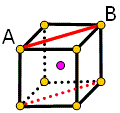(a) (b)

Figure 3138c. Example of atomic configurations if cation size decreases.

Using similar geometric calculations, we can obtain Table 3138.

Table 3138. Summary of cation-to-anion radius ratios (r+/r-) for various coordination numbers (CN).

Limiting ratio r+/r-
CN
Crystal type
Geometric shape

Material examples and their r+/r-

1.0
12 Hexagonal or cubic closest packing   Metals
0.732 - 1.0
8 Cubic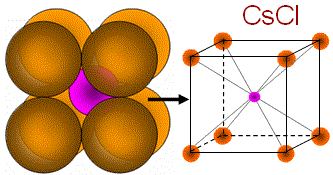(i) Binary AB: CsCl (1.13), KCl (0.91), CsBr (0.862); (ii) Binary AB2: CaF2 (0.727); (iii) Others: NH4Br
0.414 - 0.732
6 Octahedral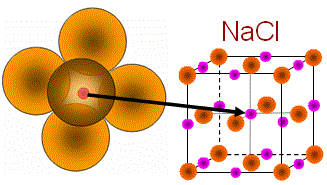(i) Binary AB: NaCl (0.70), NiAs, KBr (0.678), MgO (0.471), SrS (0.571); (ii) Binary AB2: TiO2 (0.45); (iii) Others: Al2O3
0.225 - 0.414
4 Tetrahedral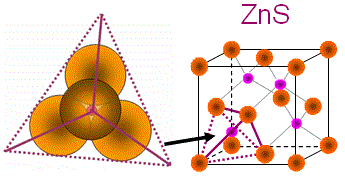(i) Binary AB: Sphalerite ((Zn,Fe)S), ZnS （0.44）, CuCl, BeS (0.35); (ii) Binary AB2: SiO2 （0.33）
0.155 - 0.225
3 Triangular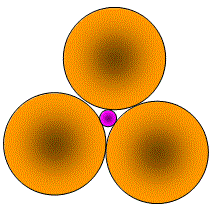CO3, NO3, BO3, B2CO3
<0.155
2 Linear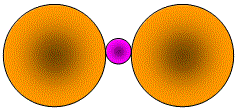UO3, CoO3, (NO2)-2

However, even though some predictions are relatively accurate (~50%) but others are not, for instance, NaCl and CsCl structures as shown in Figure 3138d.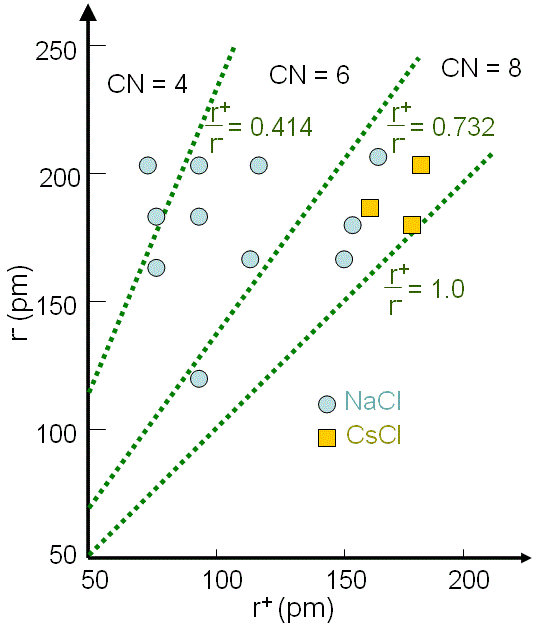Figure 3138d. Comparisons between NaCl and CsCl actual structures and their predictions by radius ratio (r+/r-) rules.

=================================================================================
The book author (Yougui Liao) welcomes your comments, suggestions, and corrections, please click here for submission. If you let book author know once you have cited this book, the brief information of your publication will appear on the “Times Cited” page.